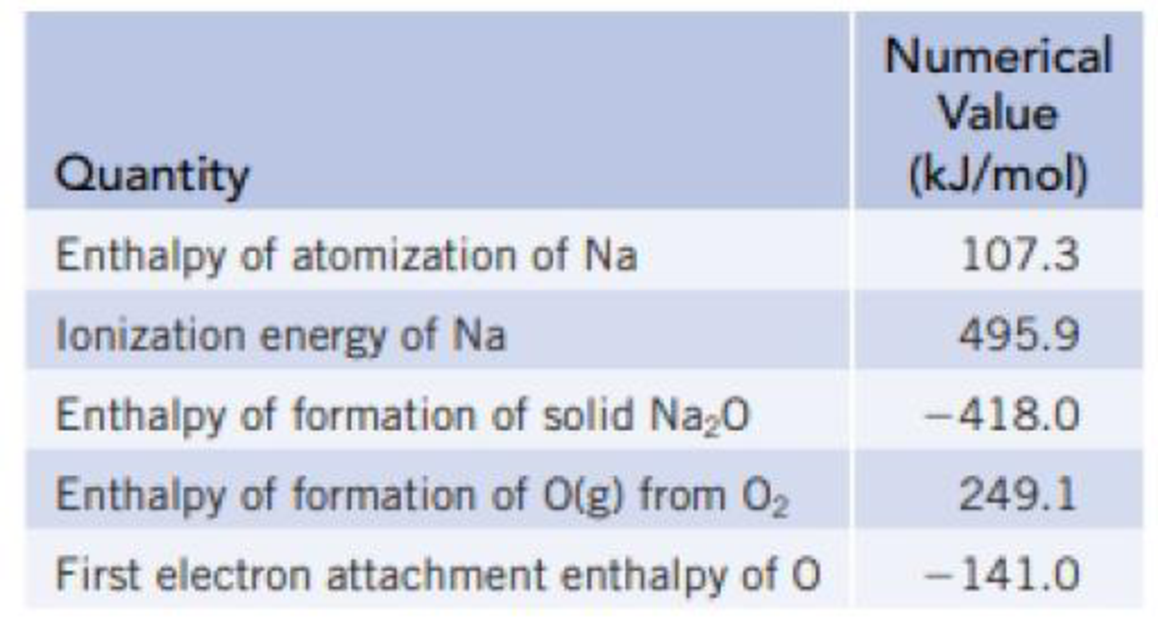Chapter 12, Problem 54GQ

Chapter
Section
Textbook Problem

Using the thermochemical data below and an estimated value of −2481 kJ/mol for the lattice energy for Na2O, calculate the value for the second electron affinity of oxygen [O−(g) + e− → O2−(g)].Interpretation Introduction

Interpretation:

The second electron affinity of the oxygen has to be calculated.

Concept introduction:

Electron affinity:

The electron affinity of an atom or molecule is defined as the amount of energy released when an electron is added to a neutral atom or molecule in the gaseous state to form a negative ion

Explanation

The second electron affinity of the oxygen is calculated as follows,

EA=ΔfHo-2ΔH at(Na)-2ΔHIE(Rb)+ΔH at(O)-ΔH at(first electron)+ΔlatticeUΔfHo=-418kJ/molΔH at(Na)=107.3kJ/molΔHIE(Rb)=495.9kJ/molΔH at(O)=249

Still sussing out bartleby?

Check out a sample textbook solution.

See a sample solution

The Solution to Your Study Problems

Bartleby provides explanations to thousands of textbook problems written by our experts, many with advanced degrees!

Get Started